Courses

# Worksheet (3): Acids, Bases and Salts Class 10 Notes | EduRev

## Class 10 : Worksheet (3): Acids, Bases and Salts Class 10 Notes | EduRev

The document Worksheet (3): Acids, Bases and Salts Class 10 Notes | EduRev is a part of the Class 10 Course Science Class 10.
All you need of Class 10 at this link: Class 10

Q.1. A compound ‘X’ of sodium is used as an antacid, and it decomposes on strong heating.
(i) Name the compound ‘X’ and give its chemical formula.
(ii) Write a balanced chemical equation to represent the decomposition of ‘X.’
(iii) Give one use of compound ‘X’ beside an antacid.  (3 Marks)
Ans.
(i) Sodium bicarbonate / Sodium hydrogen carbonate / baking soda and its formula is NaHCO3
(ii)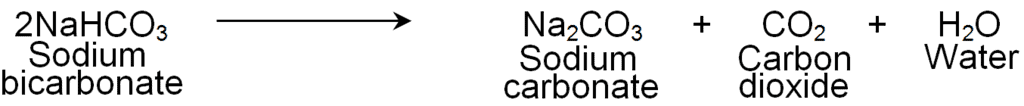(iii) It is used in fire extinguishers and for baking. (Any one)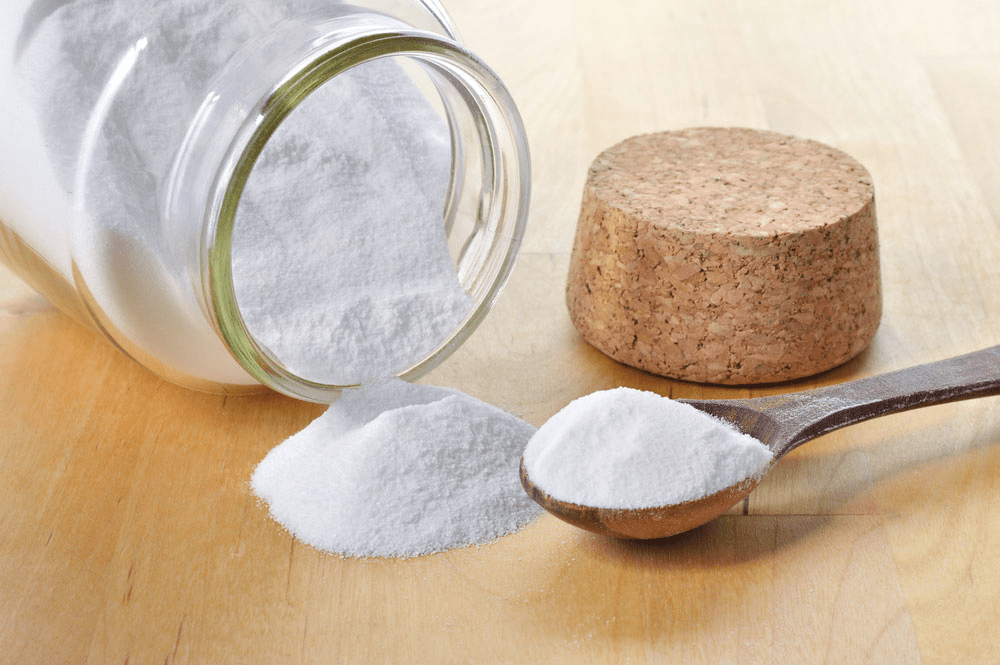Sodium Bicarbonate

Q.2.

Try yourself:Which one of the following can be used as an acid-base indicator by a visually impaired student?

Q.3. Suhana takes three beakers, A, B, and C, filled with aqueous solutions of glucose, alcohol, and hydrochloric acid, respectively, as shown in the following figure: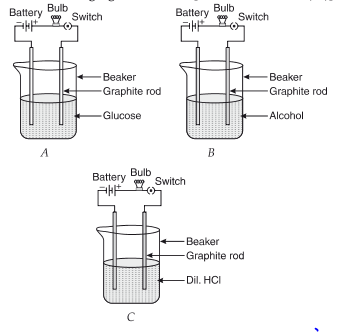(a)

Try yourself:Which of the following statements is correct in terms of glowing of bulb when the switch is ON?

(b) Justify your observations by giving a reason in each case.  (1 Marks)
Ans.
Glucose and alcohol solutions do not conduct electricity as they do not have ions. Dil. HCl contains ions, so ions' flow is responsible for the current flow.
(c)
Try yourself:Which of the following are present in a dilute aqueous solution of hydrochloric acid?

(d) Mention the change noticed with appropriate reason if beaker B's content is replaced by sodium hydroxide solution.  (1 Marks)
Ans.
After replacement, the bulb glows in B as the NaOH solution contains ions (Na+ and OH ions).

Q.4. A liquid has a pH of less than 7, representing an acidic solution.
(i) State the solution's nature if its pH increases from 7 to 14.
(ii) Mention the ion whose concentration increases with the pH value increase.
(iii) Suggest a method that is generally used for measuring the pH value.   (3 Marks)
Ans. (i) Increase in the strength of alkali. So the nature of the solution will be basic.
(ii) Hydroxide ion or hydroxyl ion or OH ion.
(iii) A paper impregnated with the universal indicator is generally used to measure a solution's pH.

Q.5.
Try yourself:Which of the following is acidic in nature?

Q.6. Study the given experimental set-up and answer the following questions.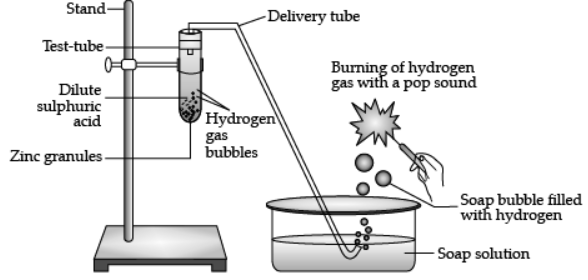(a)

Try yourself:The above experimental set up shows reaction between metal and

(b)

Try yourself:Which gas is liberated during the process?

(c) Write a balanced chemical equation for the given reaction. (1 Marks)
Ans. Zn + H2SO4 → ZnSO4 + H2
(d) What will happen if NaOH is used in place of dil. sulphuric acid, and the test tube is heated. (1 Marks)
Ans. It will produce sodium zincate (Salt) and hydrogen gas.
Zn(s) + 2NaOH(aq) → Na2ZnO2(aq) + H2(g)

Q.6. 1 g of solid sodium chloride is taken in a clean and dry test tube and 2mL of conc. Sulphuric acid is added to it. If the gas evolved is tested first with dry and then with wet blue litmus paper, will the litmus paper change color? Give a reason for your answer. What inference can be drawn about the nature of the evolved gas? Support your answer with a chemical equation for the reaction. (3 Marks)
Ans. Wet blue litmus paper
Reason: HCl produces hydrogen ions in the presence of water.

It has acidic nature.
HCl + H2O → H3O+ + Cl

Q.7.

Try yourself:One of the constituents of baking powder is sodium hydrogen carbonate; the other constituent is

Q.8. P, Q, R are different colorless solids, while S is a colorless solution. They are (in random order) Sodium chloride (NaCl), Calcium Carbonate (CaCO3), Acetic acid (CH3COOH), and Phenolphthalein indicator. Small amounts of the above substances were added in pairs (e.g., P with Q, Q with R, etc.) to a small amount of water in a test tube. They give the following results, as shown in the observation table.

Observation Table: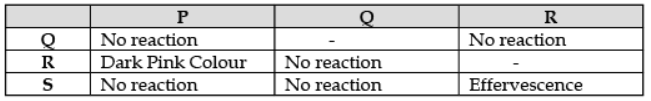(a) The chemicals are:  (1 Marks)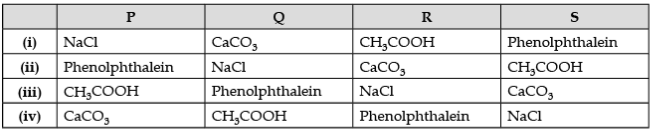(b) Write the chemical equation of the reaction involved.  (1 Marks)
(c) The chemicals that can be used as an acid-base indicator by a visually impaired student is ________. (Petunia leaves/ Vanilla essence).  (1 Marks)
(d) If acetic acid and hydrochloric acid of the same concentration are taken, which of the two is the stronger acid and why?  (1 Marks)
Ans
. (a) (ii) P - Phenolphthalein, Q – NaCl, R – CaCO3, S – CH3COOH.
(b) (i)  Phenolphthalein (P) + NaCl (Q) → No reaction
(ii)  Phenolphthalein + CaCO3 (R) → Alkaline medium (Dark Pink Colour)
(iii) CaCO3 (R) + 2CH3COOH (S) → (CH3COO)2Ca + CO2 (effervescence) + 2H2O
(c) Vanilla essence can be used as an acid-base indicator by visually impaired students as it is an olfactory indicator whose odor changes in acidic or basic media.
(d) HCl is stronger because it gives rise to more H+ ions than acetic acid.

Q.9. Assertion and Reason:
Directions: In the following questions, a statement of assertion (a) is followed by a reason (R). Mark the correct choice as:

(i)

Try yourself:Assertion (A): When zinc is added to dilute hydrochloric acid, hydrogen is given off
Reason (R): Hydrogen chloride molecules contain hydrochloric acid and hydrogen atoms.

(ii)

Try yourself:Assertion (A): To dilute concentrated sulphuric acid water is added to the acid slowly.
Reason (R): A lot of heat energy will be given out in the dilution of concentrated sulphuric acid.

(iii)

Try yourself:Assertion (A): Ammonia solution is an alkali.
Reason (R): Ammonia solution turns blue litmus paper red.

(iv)

Try yourself:Assertion (A): Gas bubbles are observed when sodium carbonate is added to dilute hydrochloric acid.
Reason (R): Carbon dioxide is given off in the reaction.

Q.10. Metal is treated with dilute sulphuric acid. The gas evolved is collected by the method shown in the figure: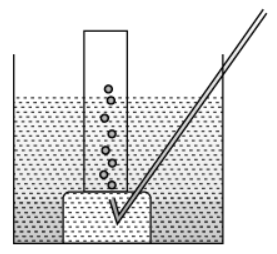(a) Name the gas. Is the gas soluble or insoluble in water? (1 Marks)
(b) Is the gaslighter or heavier than air? How will you test the gas? (1 Marks)
(c) If the metal used above is zinc, then write the chemical equation for gas evolution. (1 Marks)
(d) Write one industrial use of the gas evolved. (1 Marks)
Ans.
(a) Hydrogen gas. It is soluble in water.
(b) It is lighter than air.

Test for H2 Gas: Bring a burning matchstick near the gas jar. It burns with a pop sound.(c) Zn(s) + H2SO4(dil) → ZnSO4(aq) + H2(g)↑
(d) Liquid hydrogen is used as a fuel in rockets.

Q.11

Try yourself:Which of the following are present in a dilute aqueous solution of hydrochloric acid?

Q.12. (i) A local magician was showing magic in a village street. He took an eggshell and poured a solution over it. As a result, effervescence was formed. When he took a burning matchstick over it, it went off:
(a) Identify the solution poured and the substance present in the eggshell.
(b) What is the reason behind effervescence?
(c) Write its balanced chemical equation.
(d) Give the common name of the substance present in the eggshell.
(ii) Draw a labeled diagram to show that acid solution in water conducts electricity.  (5 Marks)
Ans.
(i) (a) Solution is an acid. The substance in eggshells is calcium carbonate.
(b) Effervescence is due to the evolution of CO2 gas.
(c) 2HNO3 + CaCO→ Ca(NO3)2 + H2O + CO2
(d) Limestone.
(ii)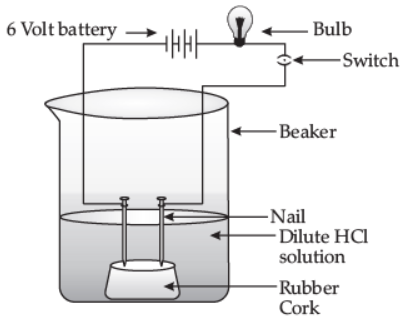An acid solution in water conducts electricity.

Q.13.

Try yourself:Which of the following salts does not contain water of crystallisation?

Q.14. Write balanced chemical equations for the following statements : (5 Marks)(i) Bleaching powder is kept open in the air.
(ii) Blue crystals of copper sulfate are heated.
(iii) Chlorine gas is passed through dry slaked lime.
(iv) Carbon dioxide gas is passed through lime water.
(v) NaOH solution is heated with zinc granules.
Ans. (i) CaOCl2(s) + CO2(g) → CaCO3(s) + Cl2(g)

(Bleaching powder) (Carbon     (Calcium     (Chlorine)

dioxide)    carbonate)
(iii) Ca(OH)2  + Cl2 → CaOCl2 + H2O

(Dry slaked lime) (Chlorine)  (Bleaching powder)
(iv) Ca(OH)2 + CO2 → CaCO3 + H2O

(Lime water)            (Calcium Carbonate) (Milky)

Sanjana, while preparing the cake, used baking soda in small amounts. It helps to make the cake soft and spongy. An aqueous solution of baking soda also turns red litmus blue. It is also used in soda acid extinguishers.
(a) How does baking soda help in extinguishing fire? (1 Marks)
(b) Write the chemical reaction involved when it is heated. (1 Marks)
(c) Is the pH value of baking soda solution lower than or higher than 7? (1 Marks)
(d) An aqueous solution turns the red litmus solution blue. Excess addition of which solution would reverse the change? (1 Marks)
Ans.
(a) The CO2 gas produced by baking soda and acid in the soda – acid fire extinguisher helps in extinguishing the fire.
2NaHCO3 (s) + H2SO4 (aq) → Na2SO4 (s) + 2H2O (l) + 2CO2 (g)
(b) When it is heated, it disintegrates into sodium carbonate, water, and carbon dioxide.
2NaHCO3 (s) → Na2CO(s) + H2O (l) + CO2 (g)
(c) The baking soda solution's pH value is higher than 7, i.e., it is alkaline.
(d) The given solution is alkaline as it changes red litmus blue. When acid is added to the given alkaline solution, it neutralizes the base, and further addition of acid makes the solution acidic that turns blue litmus red.

Q.16

Try yourself:Common salt, besides being used in the kitchen can also be used as the raw material for making
(i) washing soda.
(ii) bleaching powder.
(iii) baking soda.
(iv) slaked lime.

A dry pellet of a common base B, when kept in open, absorbs moisture and turns sticky.
The compound is also a by-product of the Chlor-alkali process.
(a) Identify B.  (1 Marks)
(b) What type of reaction occurs when B is treated with an acidic oxide?   (1 Marks)
(c) Write a balanced chemical equation for one such solution.   (1 Marks)
(d) When aqueous sodium carbonate (Na2CO3) reacts with HCl (aq), it gives   (1 Marks)
(i) NaOH, H2 and CO2
(ii) NaCl, H2O and CO2
(iii) NaHCO3, H2 and CO2
(iv) NaHCO3, H2O and CO2
Ans.
(a) B is sodium hydroxide (NaOH).
(b) Neutralisation reaction occurs when B is treated with an acidic oxide.
(c) 2NaOH (s) + CO2 (g) → Na2CO3 (s) + H2O (l)
(d) (ii) NaCl, H2O and CO2
Na2CO3(aq) + 2HCl(aq) → 2NaCl + H2O + CO2

Q.18.

Try yourself:One of the constituents of baking powder is sodium hydrogen carbonate; the other constituent is

Q.19. Assertion and Reason :
Directions: In the following questions, a statement of assertion (A) is followed by a statement of reason (R). Mark the correct choice as :
(i)
Try yourself:Assertion (A): When common salt is kept open, it absorbs moisture from the air.
Reason (R): Common salt contains magnesium chloride.

(ii)

Try yourself:Assertion (A): Baking soda creates acidity in the stomach.
Reason (R): Baking soda is alkaline.

(iii)

Try yourself:Assertion (A): Plaster of Paris is used by doctors by setting fractured bones.
Reason (R): When Plaster of Paris is mixed with water and applied around the fractured limbs, it sets into a hard mass.

Q.20 (i) Define universal indicator. For what purpose is it used?
(ii) Two solutions, A and B, have pH values of 3.0 and 9.5, respectively. Which of these will turn litmus solution from blue to red and turn phenolphthalein from colorless to pink?
(iii) Water is a neutral substance. What color will you get when you add a few drops of universal indicator to a test tube containing distilled water?  (5 Marks)
Ans. (i) A universal indicator is a mixture of many different indications (or dyes) which give different colors at different pH values of the entire pH scale. The color produced by the universal indicator is used to find the pH value of acid or base by matching the color with the colors on the pH color chart.
(ii) Solution A is acidic and will turn the litmus solution from blue to red.
Solution B is basic and will turn phenolphthalein from colorless to pink.
(iii) Green color will be obtained.

Offer running on EduRev: Apply code STAYHOME200 to get INR 200 off on our premium plan EduRev Infinity!

## Science Class 10

80 videos|257 docs|114 tests

,

,

,

,

,

,

,

,

,

,

,

,

,

,

,

,

,

,

,

,

,

,

,

,

;